Processing ......FreeComputerBooks.com Links to Free Computer, Mathematics, Technical Books all over the World

Probability Theory and Stochastic Process
Related Book Categories:
 Bayesian Thinking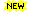Machine LearningThe R Programming Financial Mathematics and Engineering Applied Mathematics
•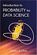Introduction to Probability for Data Science (Stanley Chan)

This book is an introductory textbook in undergraduate probability in the context of data science to emphasize the inseparability between data (computing) and probability (theory) in our time, with examples in both MATLAB and Python.

•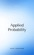Applied Probability (Paul Pfeiffer)

This book presents a unique blend of theory and applications, with special emphasis on mathematical modeling, computational techniques, and examples from the real world application in industries and science.

•Introduction to Probability, Statistics, and Random Processes

This book introduces students to probability, statistics, and stochastic processes. It provides a clear and intuitive approach to these topics while maintaining mathematical accuracy. The book contains a large number of solved exercises.

•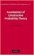Foundations of Constructive Probability Theory (Yuen-Kwok Chan)

This book provides a systematic and general theory of probability within the framework of Constructive Mathematics. It can serve as a parallel introduction into constructive mathematics and rigorous probability theory.

•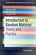Introduction to Random Matrices: Theory and Practice

This is a book for absolute beginners. The aim is to provide a truly accessible introductory account of Random Matrix theory. Most chapters are accompanied by MATLAB codes to guide readers through the numerical check of most analytical results.

•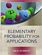Elementary Probability for Applications (Rick Durrett)

This clear and lively introduction to probability theory concentrates on the results that are the most useful for applications, including combinatorial probability and Markov chains. Concise and focused, it is designed for students familiar with basic calculus.

•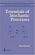Essentials of Stochastic Processes (Rick Durrett)

Stochastic processes have become important for many fields, including mathematical finance and engineering. Written by one of the worlds leading probabilists, this book presents recent results previously available only in specialized monographs.

•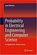Probability in Electrical Engineering and Computer Science

This textbook motivates and illustrates the techniques of applied probability by applications in electrical engineering and computer science (EECS). Python labs enable the readers to experiment and consolidate their understanding.

•Introduction to Probability (Charles M. Grinstead, et al)

The book is a beautiful introduction to probability theory at the beginning level. The book contains a lot of examples and an easy development of theory without any sacrifice of rigor, keeping the abstraction to a minimal level.

•Probability Theory: The Logic of Science (E. T. Jaynes)

Going beyond the conventional mathematics of probability theory, this study views the subject in a wider context. It discusses new results, along with applications of probability theory to a variety of problems.

•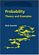Probability: Theory and Examples (Rick Durrett)

This lively introduction to measure-theoretic probability theory covers laws of large numbers, central limit theorems, random walks, martingales, Markov chains, ergodic theorems, and Brownian motion.

•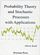Probability Theory and Stochastic Processes with Applications

This book provides an introduction to probability theory and discrete and continuous stochastic processes and its applications. It has a unique approach that provides a broad and wide introduction into the fascinating area of probability theory.

•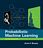Probabilistic Machine Learning: An Introduction (Kevin Murphy)

This book is a comprehensive introduction to machine learning that uses probabilistic models and inference as a unifying approach. It is written in an informal, accessible style, complete with pseudo-code for the most important algorithms.

•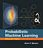Probabilistic Machine Learning: Advanced Topics (Kevin Murphy)

This book expands the scope of Machine Learning to encompass more challenging problems, discusses methods for discovering 'insights' about data, and how to use probabilistic models for causal inference and decision making under uncertainty.

•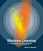Machine Learning: A Probabilistic Perspective (Kevin Murphy)

This textbook offers a comprehensive and self-contained introduction to the field of machine learning, based on a unified, probabilistic approach. It is written in an informal, accessible style, complete with pseudo-code for the most important algorithms.

•Seeing Theory: A Visual Introduction to Probability and Statistics

The goal of this book is to make statistics more accessible through interactive visualizations (using D3.js). It visualizes the fundamental concepts covered in an introductory college statistics or Advanced Placement statistics class.

•Measure, Integration and Real Analysis (Sheldon Axler)

This textbook welcomes students into the fundamental theory of measure, integration, and real analysis. Focusing on an accessible approach, it lays the foundations for further study by promoting a deep understanding of key results.

•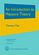An Introduction to Measure Theory (Terrence Tao)

This is a graduate text introducing the fundamentals of measure theory and integration theory, which is the foundation of modern real analysis, intended to cover a quarter or semester's worth of material for a first graduate course in real analysis.

•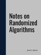Notes on Randomized Algorithms (James Aspnes)

This book introduces the basic concepts in the design and analysis of randomized algorithms. Discusses tools from probability theory, including random variables and expectations, union bound arguments, concentration bounds, martingales, etc.

•Stochastic Differential Equations: Models and Numerics

The goal of this book is to give useful understanding for solving problems formulated by stochastic differential equations models in science, engineering and mathematical finance. Typically, these problems require numerical methods to obtain a solution.

•Basic Probability Theory (Robert B. Ash)

This introduction book emphasizes the probabilistic way of thinking, surveys random variables, conditional probability and expectation, characteristic functions, infinite sequences of random variables, Markov chains, and an introduction to statistics.

•Applied Probability (Paul E. Pfeiffer)

In addition to an introduction to the essential features of basic probability in terms of a precise mathematical model, the work describes and employs user defined MATLAB procedures and functions to solve many important problems in basic probability.

•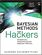Bayesian Methods for Hackers: Probabilistic Programming

This book illuminates Bayesian inference through probabilistic programming with the powerful PyMC language and the closely related Python tools NumPy, SciPy, Matplotlib, through practical examples and computation - no advanced mathematics required.

•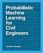Probabilistic Machine Learning for Civil Engineers

This comprehensive textbook presents basic machine learning methods for civil engineers who do not have a specialized background in statistics or in computer science. It includes several case studies that students and professionals will appreciate.

•Probability on Trees and Networks (Russell Lyons, et al)

This book is concerned with certain aspects of discrete probability on infinite graphs that are currently in vigorous development. Of course, finite graphs are analyzed as well, but usually with the aim of understanding infinite graphs and networks.

•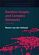Random Graphs and Complex Networks (Remco van der Hofstad)

This rigorous introduction to network science presents Random Graphs as models for real-world networks. Such networks have distinctive empirical properties and a wealth of new models have emerged to capture them.

•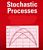Advanced Stochastic Processes (David Gamarnik)

This guide to Stochastic Processes covers a wide range of topics. Short, readable chapters aim for clarity rather than full generality. The objectives of the text are to introduce students to the standard concepts and methods of stochastic modeling

•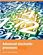Advanced Stochastic Processes (Jan A. Van Casteren)

This comprehensive guide to Stochastic Processes gives a complete overview of the theory and addresses the most important applications. Basically self-contained, it is both a course book and a rich resource for individual readers.

•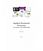Applied Stochastic Processes in Science and Engineering

This book introduces modern concepts of applied stochastic processes is written for a broad range of applications in diverse areas of engineering and the sciences. Written for a senior undergraduate course offered to students with a suitably mathematical background.

•Probability and Statistics: A Course for Physicists and Engineers

This book offers an introduction to concepts of probability theory, probability distributions relevant in the applied sciences, and focuses on real engineering applications, as well as basics of sampling distributions, estimation and hypothesis testing.

•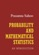Probability and Mathematical Statistics (Prasanna Sahoo)

This book presents an introduction to probability and mathematical statistics for students already having some elementary mathematical background. It blends proven coverage with new innovations to ensure you gain a solid understanding of statistical concepts.

•StatLect - Lectures on Probability Theory and Statistics

This is a collection of lectures on probability theory and mathematical statistics. It is offered as a free service to the mathematical community and provides an accessible introduction to topics that are not usually found in elementary textbooks.

•Probabilistic Programming and Bayesian Methods for Hackers

This book is designed as an introduction to Bayesian inference from a computational understanding-first, and mathematics-second, point of view. The book assumes no prior knowledge of Bayesian inference nor probabilistic programming.

•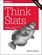Think Stats, 2nd Edition: Exploratory Data Analysis in Python

This concise introduction shows you how to perform statistical analysis computationally, rather than mathematically, with programs written in Python.

•Gaussian Processes for Machine Learning (Carl E. Rasmussen)

This book provides a long-needed systematic and unified treatment of theoretical and practical aspects of Gaussian Processes (GPs) in machine learning. It deals with the supervised-learning problem for both regression and classification.

•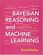Bayesian Reasoning and Machine Learning (David Barber)

This practical introduction is ideally suited to computer scientists without a background in calculus and linear algebra. You'll develop analytical and problem-solving skills that equip them for the real world. Numerous examples and exercises are provided.

•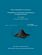From Algorithms to Z-Scores: Probabilistic and Statistical Modeling

This is a textbook for a course in mathematical probability and statistics for computer science students. Computer science examples are used throughout, in areas such as: computer networks; data and text mining; computer security; etc.

•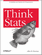O'Reilly® Think Stats: Probability and Statistics for Programmers

This book shows you how to perform statistical analysis computationally, rather than mathematically, with programs written in Python.

•Stochastic Modeling and Control (Ivan Ganchev Ivanov)

The book provides a self-contained treatment on practical aspects of stochastic modeling and calculus including applications drawn from engineering, statistics, and computer science.

•Discrete-Event Control of Stochastic Networks (Eitan Altman, et al)

Opening new directions in research in both discrete event dynamic systems as well as in stochastic control, this volume focuses on a wide class of control and of optimization problems over sequences of integer numbers.

•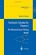Stochastic Calculus and Finance (Steven E. Shreve)

The book gives both precise statements of results, plausibility arguments, and even some proofs, but more importantly intuitive explanations developed and refine through classroom experience with this material are provided.

•Probability, Geometry and Integrable Systems (Mark Pinsky, et al)

The three main themes of this book, probability theory, differential geometry, and the theory of integrable systems, reflect the broad range of mathematical interests of Henry McKean, to whom it is dedicated.

•Introduction to Probability and Statistics Using R (G. Jay Kerns)

This is a textbook for an undergraduate course in probability and statistics. The approximate prerequisites are calculus and some linear algebra. Students attending the class include mathematics, engineering, and computer science majors.

•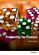Probability for Finance (Patrick Roger)

This book provides technical support for students in finance. It reviews the main probabilistic tools used in financial models in a pedagogical way, starting from simple concepts like random variables and tribes and going to more sophisticated ones like conditional expectations and limit theorems.

•Stochastic Processes for Finance (Patrick Roger)

It describes the most important stochastic processes used in finance in a pedagogical way, especially Markov chains, Brownian motion and martingales. It also shows how mathematical tools like filtrations, Ito's lemma or Girsanov theorem should be understood in the framework of financial models.

•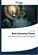Basic Queueing Theory: System Performance Modeling

Queueing Theory is one of the most commonly used mathematical tool for the performance evaluation of systems. The aim of the book is to present the basic methods, approaches in a Markovian level for the analysis of not too complicated systems.

•Theory and Applications of Monte Carlo Simulations (Wai Kin Chan)

The purpose of this book is to introduce researchers and practitioners to recent advances and applications of Monte Carlo Simulation (MCS). Random sampling is the key of the MCS technique.

•Lists, Decisions and Graphs - With an Introduction to Probability

In this book, four basic areas of discrete mathematics are presented: Counting and Listing (Unit CL), Functions (Unit Fn), Decision Trees and Recursion (Unit DT), and Basic Concepts in Graph Theory (Unit GT).

Book Categories
 :All CategoriesRecent BooksMiscellaneous BooksComputer LanguagesComputer ScienceData Science/DatabasesElectrical EngineeringJava and Java EE (J2EE)Linux and UnixMathematicsMicrosoft and .NETMobile ComputingNetworking and CommunicationsSoftware EngineeringSpecial TopicsWeb Programming
Other Categories